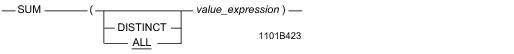# 15.00 - SUM - Teradata Database

## Teradata Database SQL Functions, Operators, Expressions, and Predicates

Product
Release Number
15.00
Content Type
Programming Reference
Publication ID
B035-1145-015K
Language
English (United States)

## SUM

### Purpose

Returns a column value that is the arithmetic sum of value_expression.

### Syntaxwhere:

 Syntax element … Specifies … ALL that all non-null values specified by value_expression, including duplicates, are included in the sum computation for the group. This is the default. DISTINCT that duplicate and non-null values specified by value_expression are eliminated from the sum computation for the group. value_expression a literal or column expression for which the sum is to be computed. The expression cannot contain any ordered analytical or aggregate functions.

### ANSI Compliance

This is ANSI SQL:2011 compliant.

### Result Type and Attributes

The following table lists the default attributes for the result of SUM(x).

 Data Type of Operand Data Type of Result Format Title BYTEINT or SMALLINT Same as the operand Default format of the INTEGER data type Sum(x) character Same as the operand Default format for FLOAT UDT Same as the operand Format for the data type to which the UDT is implicitly cast DECIMAL(n,m) DECIMAL(p,m), where p is determined by the rules in the following rules: If MaxDecimal in DBSControl is 0 or 15 and n ≤ 15, then p = 15. 15 < n ≤ 18, p = 18. n > 18, then p = 38. If MaxDecimal in DBSControl is 18 and n ≤ 18, then p = 18. n > 18, then p = 38. If MaxDecimal in DBSControl is 38 and n = any value, the p = 38. Default format for the data type of the operand Sum(x) Other than UDT, SMALLINT, BYTEINT, DECIMAL, or character Same as the operand Default format for the data type of the operand

For an explanation of the formatting characters in the format, and information on data type default formats, see “Data Type Formats and Format Phrases” in SQL Data Types and Literals.

### Support for UDTs

By default, Teradata Database performs implicit type conversion on a UDT argument that has an implicit cast that casts between the UDT and either of the following predefined types:

• Numeric
• Character
• To define an implicit cast for a UDT, use the CREATE CAST statement and specify the AS ASSIGNMENT clause. For more information on CREATE CAST, see SQL Data Definition Language.

Implicit type conversion of UDTs for system operators and functions, including SUM, is a Teradata extension to the ANSI SQL standard. To disable this extension, set the DisableUDTImplCastForSysFuncOp field of the DBS Control Record to TRUE. For details, see Utilities: Volume 1 (A-K).

For more information on implicit type conversion of UDTs, see Chapter 13: “Data Type Conversions.”

### Usage Notes

If value_expression is a column reference, the column must not be to a view column that is derived from a function.

SUM is valid only for numeric data.

Nulls are not included in the result computation. For details, see “Manipulating Nulls” in SQL Fundamentals and “Aggregates and Nulls” on page 31.

The SUM function can result in a numeric overflow or the loss of data because of the default output format. If this occurs, a data type declaration may be used to override the default.

For example, if QUANTITY comprises many rows of INTEGER values, it may be necessary to specify a data type declaration like the following for the SUM function:

`   SUM(QUANTITY(FLOAT))`

### Possible Result Overflow with SELECT Sum

When using this function, the result can create an overflow when the data type and format are not in sync. For a column defined as:

`Salary Decimal(15,2) Format ‘\$ZZZ,ZZ9.99’`

The following query:

`SELECT SUM (Salary) FROM Employee;`

causes an overflow because the decimal operand and the format are not in sync.

To avoid possible overflows, explicitly specify the format for decimal sum to specify a format large enough to accommodate the decimal sum resultant data type.

`SELECT Sum(Salary) (format ‘\$Z,ZZZ,ZZZ,ZZ9.99) FROM Employee;`

### SUM Window Function

For the SUM function that returns the cumulative, group, or moving sum, see “Window Aggregate Functions” on page 984.

### Example : Accounts Receivable

You need to know how much cash you need to pay all vendors who billed you 30 or more days ago.

`   SELECT SUM(Invoice)`
`   FROM AcctsRec`
`   WHERE (CURRENT_DATE - InvDate) >= 30;`

### Example : Face Value of Inventory

You need to know the total face value for all items in your inventory.

`   SELECT SUM(QUANTITY * Price)`
`   FROM Inventory;`
`   `
`   Sum((QUANTITY * Price))`
`   -----------------------`
`             38,525,151.91`